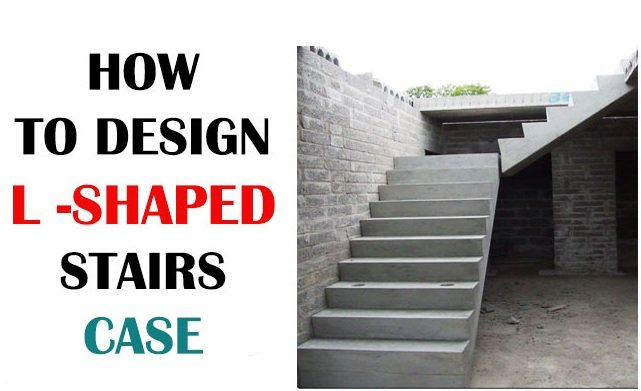Breaking News
Home / Civil Engineering / How to design L Shaped Stairs Case

# How to design L Shaped Stairs Case

## How to design L Shaped Stairs Case

If the shape of the stair is like L, it is called as L type stair. In this type of stair, the angle remains 90 degree. This type of stair is supported with landing & two walls in ground level and first-floor level. Height from the plinth level to the first floor should be retained.There is a need for a riser.

Maximum riser size = 7” (inch)

Minimum riser size = 4” (inch)

Maximum tread size = 12” (inch)

Minimum tread size = 10” (inch)

Landing is taken as = 3’ 3”

Tread is taken as = 10”

Riser taken as = 7”

### What Is Estimation and Costing

10 ‘ 6 “height is equivalent to 10.5 inches

Riser is 7″/12 = 0.5833 foot [ 1 feet = 12 inches ]

Tread is 0.8333 feet equal to 10″/12

The number of steps identical to height/riser = 10.5/0.5833 = 18 steps

The length of the landing is 3 ‘ 3 “equal to 3.25 feet

The width is 3 ‘ 3 “= 3.25 ft

Length of stairs is identical to the total stairs-1 = 18-1 = 17

Overall treads = 17 – Landing = 16

Total length of the tread = 16 x 0.8333 [ one tread ] = 13.3328 ft

Landing involves = tread length + landing length + width = 13.3328 + 3.25 + 3.25 = maximum stair length = 19.8328 ft.### THANKS.